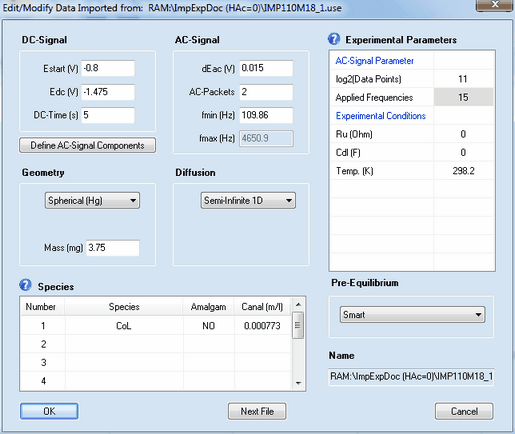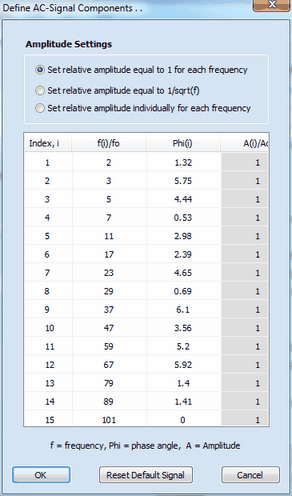﻿ Menu Command: Edit/Modify
DigiElch Help

# Menu Command: Edit/Modify# Menu Command: Edit/ModifyThis command opens a dialog box that enables the user to view (or modify) the parameters imported from an experimental data file.Most of the parameters have exactly the same meaning as already described for cyclic voltammetry. The meaning and functionality of the remaining parameter is as follows:

## 1. DC - Signal

Estart (V), Edc (V), DC-Time (s)
The impedance data are assumed to refer to an experiment executed in the following way: at the beginning of the experiment the potential of the electrode is assumed to be at Estart (V) so that  the initial concentrations are in agreement with the Pre-Equilibrium setting computed for this potential. Then the electrode potential jumps to Edc (V) and remains at this value for the time indicated by DC-Time (s) before the AC-Signal is superimposed.

## 2. AC-Signal Parameters

log2(Data Points)
The number of data points used for generating the AC-signal. It must be an integer power of 2 and is 2^11 = 2048 by default.

Applied Frequencies
Number of  frequency components from which the multi-sine AC-signal is generated.

## 3. AC - Signal

dEac (V), AC-Packets, fmin (Hz), fmax (Hz)
The AC-Signal can be composed of up to 15 frequencies components which can be edited/defined by clicking on the button Define AC-Signal ComponentsThe AC-signal is assumed to be composed of up to 15 frequency components defined by frequency, fi,  phase angle, φi, and amplitude, Ai.. The values for frequency and amplitude are relative values referring to the basic frequency, fo  (i.e. the frequency which would be obtained if the signal formed by the 2n data points (where n is the number defined in log2(Data Points)) covers exactly a single sinus cycle.) The overall AC-Signal is generated using the number of frequency components defined in Applied Frequencies. Then the amplitude of the overall signal is adjusted such as to mach the value defined in dEac (V) and the time regime is adjusted in such a way that the lowest frequency component is equal to fmin (Hz). The "real world" value of the  highest frequency component, fmax (Hz), depends on the number of Applied Frequencies and the value entered for fmin (Hz). The overall AC-Signal is cyclically applied n-times where n is the number defined in AC-Packets. Consequently, AC-Packets = 2 means that the overall signal is applied twice in the course of the simulation while the Fourier-Transformation is always executed for the last of the applied AC-Packets.
It is assumed the experiment starts with jumping the potential from Estart (V) to Edc (V). The potential remains at this value for the time interval given by DC-Time(s). Then the AC-Signal is repeatedly superimposed where the number of repetitions is given by AC-Packets. The impedance data are computed from the current referring to the last AC-Packet and the applied electrode potential.